# GMAT Math : DSQ: Calculating the perimeter of a square

## Example Questions

### Example Question #177 : Data Sufficiency Questions

Gene is building a fence. He is using square fence posts and needs to know the total distance around one pole. Help him find the distance,

I) The fence will befeet tall andfeet long.

II) The diagonal distance from one corner of a fence post to its other corner is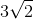inches.

Either statement is sufficient to answer the question.

Statement I is sufficient to answer the question, but statement II is not sufficient to answer the question.

Statement II is sufficient to answer the question, but statement I is not sufficient to answer the question.

Both statements are needed to answer the question.

Statement II is sufficient to answer the question, but statement I is not sufficient to answer the question.

Explanation:

We are asked to find the perimeter of a square. To do that we need a side length.

I) Is irrelevant and is trying to distract you with other aspects of the question.

II) Gives you the diagonal of the square. The diagonal of a square creates two 45/45/90 triangles.

Use this knowledge to find the other side lengths and then the perimeter.

### Example Question #178 : Data Sufficiency Questions

Find the perimeter of the square.

1. The length of the diagonal of the square is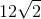.
2. The area of the square is.

Statement 1 alone is sufficient, but statement 2 alone is not sufficient to answer the question.

Statements 1 and 2 are not sufficient, and additional data is needed to answer the question.

Each statement alone is sufficient to answer the question.

Both statements taken together are sufficient to answer the question, but neither statement alone is sufficient.

Statement 2 alone is sufficient, but statement 1 alone is not sufficient to answer the question.

Each statement alone is sufficient to answer the question.

Explanation:

Statement 1: The length of the diagonal of a square is found by multiplying the length of one side by the square root of. In this case, it is easy to see the length of the side is.

Knowing the length of the side, we can find the perimeter of the square: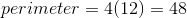Statement 2: We can use the given area to solve for the length of the side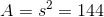whererepresents the side's length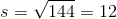We can now find the perimeter in the same manner:Therefore, each statement alone is sufficient to answer the question.

### Example Question #179 : Data Sufficiency Questions

The ratio of square A to square B is 3:1. Find the perimeter of square B

1. The area of square is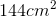.
2. The length of the diagonal of square B is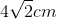.

Both statements taken together are sufficient to answer the question, but neither statement alone is sufficient.

Statement 2 alone is sufficient, but statement 1 alone is not sufficient to answer the question.

Statement 1 alone is sufficient, but statement 2 alone is not sufficient to answer the question.

Each statement alone is sufficient to answer the question.

Statements 1 and 2 are not sufficient, and additional data is needed to answer the question.

Each statement alone is sufficient to answer the question.

Explanation:

Statement 1: We can use the area to find the length of square A's side.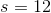Keep in mind that the ratio of square A to square is 3:1.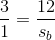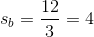Now that we know the length of the side, we can find the perimeter of square B.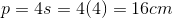Statement 2: We can use the diagonal to find the length of the side.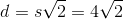We can easily see the side measures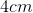so we can now find the perimeter.### Example Question #180 : Data Sufficiency Questions

What is the perimeter of the square?

1. A side measures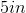.
2. The area of the square is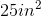.

Statements 1 and 2 are not sufficient, and additional data is needed to answer the question.

Statement 2 alone is sufficient, but statement 1 alone is not sufficient to answer the question.

Statement 1 alone is sufficient, but statement 2 alone is not sufficient to answer the question.

Both statements taken together are sufficient to answer the question, but neither statement alone is sufficient.

Each statement alone is sufficient to answer the question.

Each statement alone is sufficient to answer the question.

Explanation:

Recall the formula for perimeter of a square: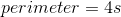whererepresents the length of a side.

Statement 1: We're given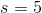so we can find the perimeter: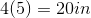Statement 2: We're given the area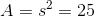so we can solve for.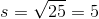With, we can calculate the perimeter:Each statement alone is sufficient to answer the question.

### Example Question #21 : Squares

Find the perimeter of the square.

1. The diagonal measures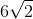inches.
2. The diagonal is found by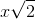whererepresents a square's side length.

Each statement alone is sufficient to answer the question.

Both statements taken together are sufficient to answer the question, but neither statement alone is sufficient.

Statements 1 and 2 are not sufficient, and additional data is needed to answer the question.

Statement 2 alone is sufficient, but statement 1 alone is not sufficient to answer the question.

Statement 1 alone is sufficient, but statement 2 alone is not sufficient to answer the question.

Statement 1 alone is sufficient, but statement 2 alone is not sufficient to answer the question.

Explanation:

Statement 1: We can find the length of the square's side using the information given.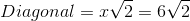so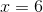We can now find the perimeter of the square: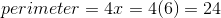inches

Statement 2: In order to find the length of the square's side using the information provided in Statement 1, we need to use this equation.

Statement 1 alone is sufficient, but statement 2 alone is not sufficient to answer the question.

Tired of practice problems?

Try live online GMAT prep today.